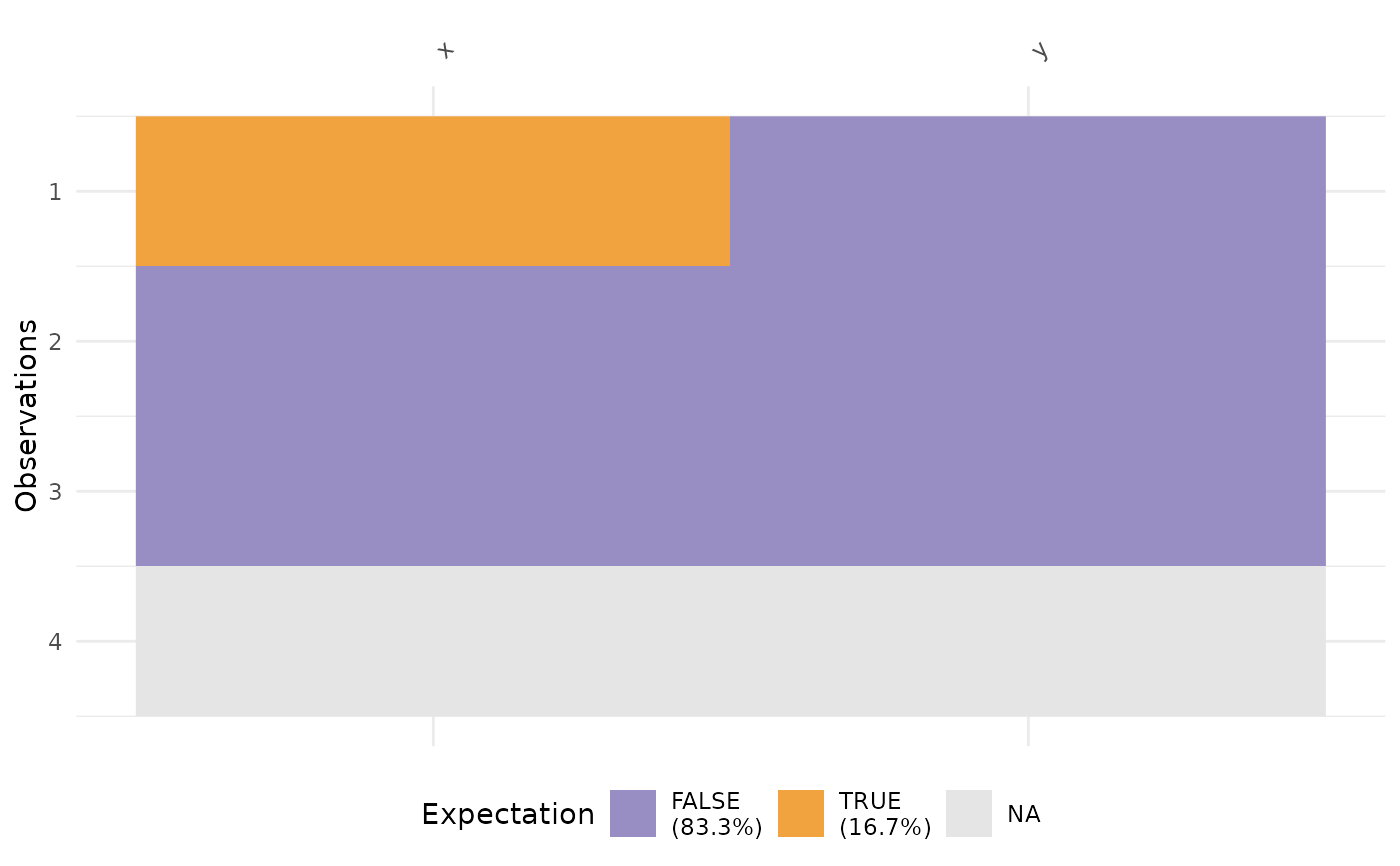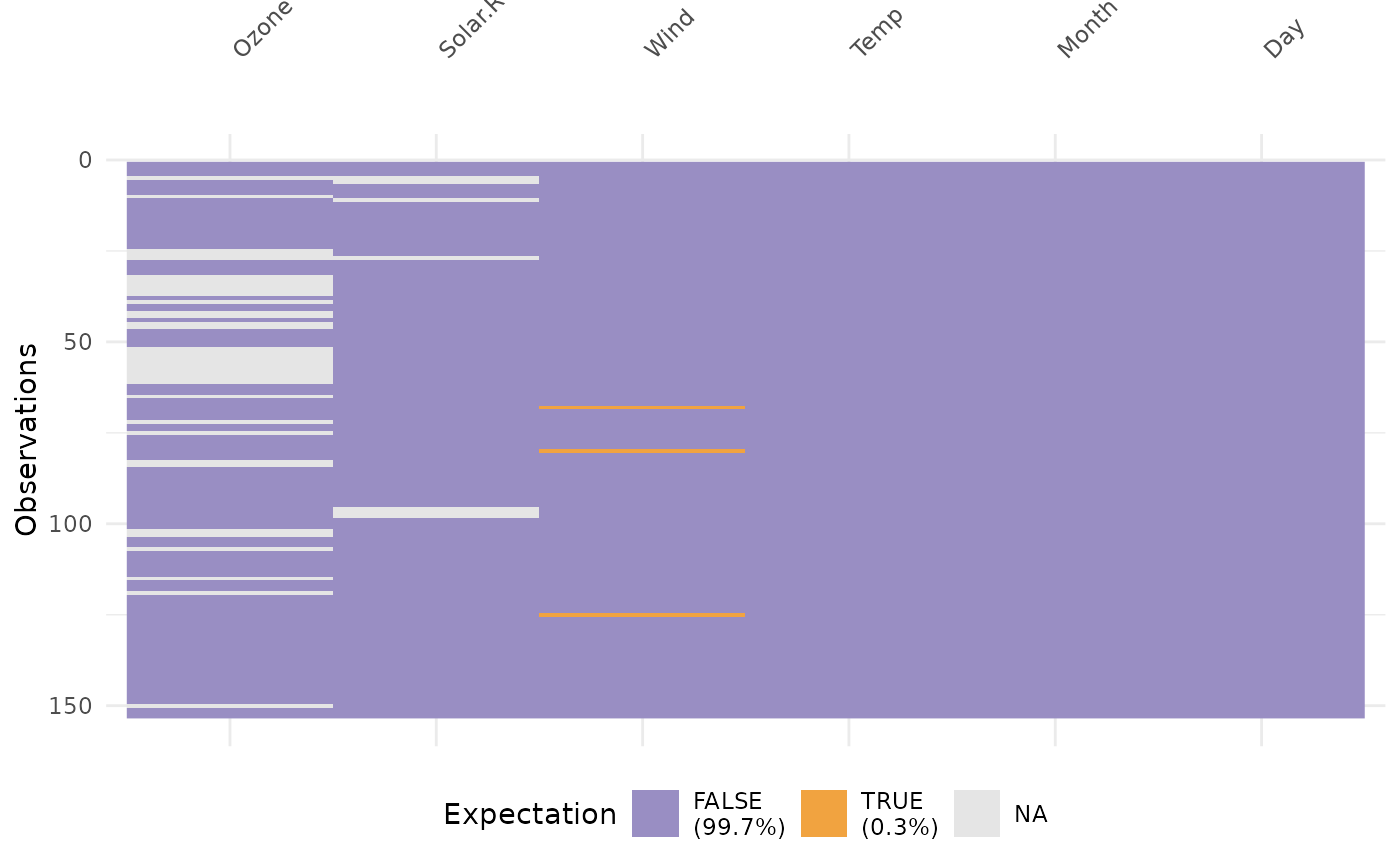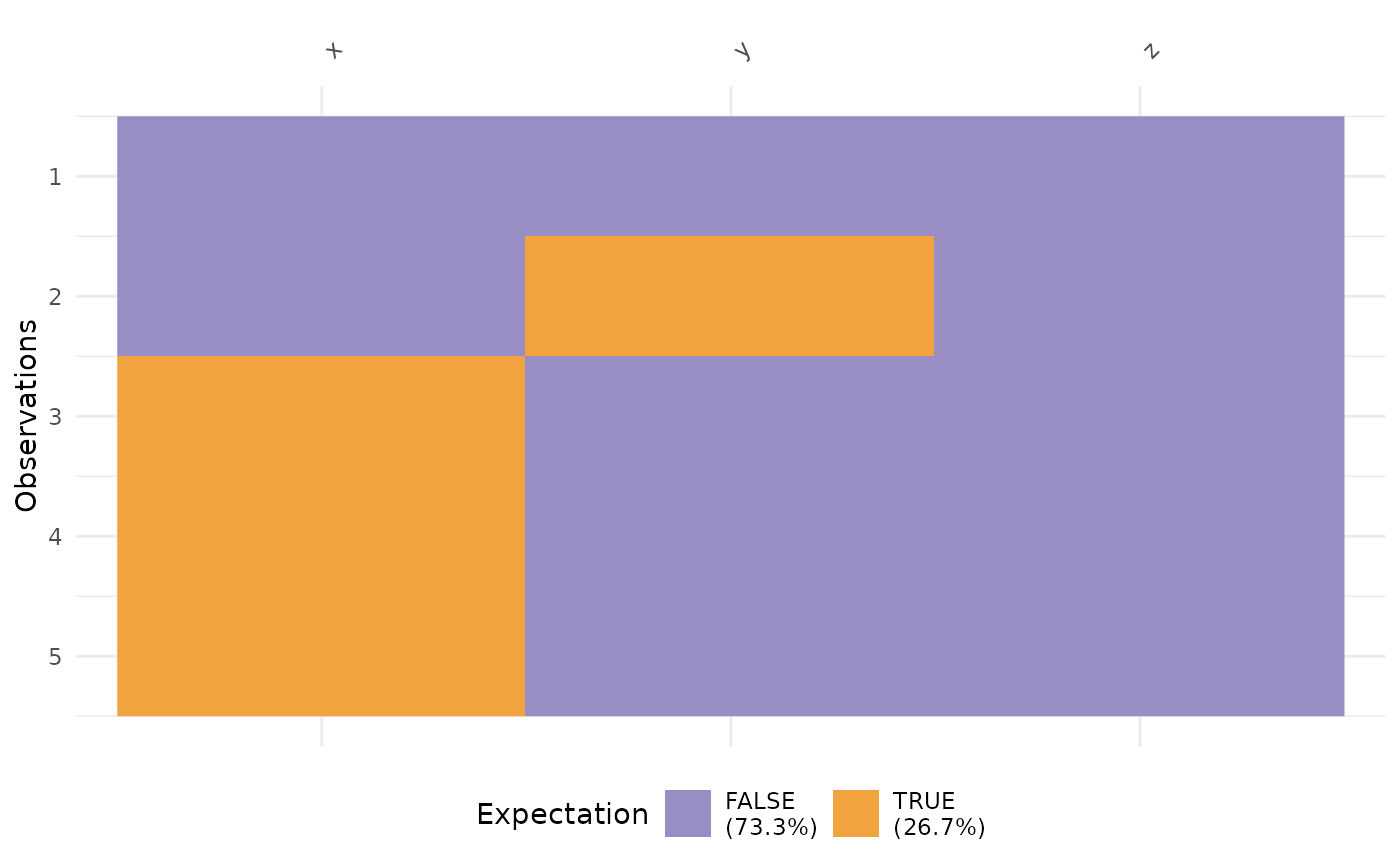`vis_expect` visualises certain conditions or values in your data. For example, If you are not sure whether to expect -1 in your data, you could write: `vis_expect(data, ~.x == -1)`, and you can see if there are times where the values in your data are equal to -1. You could also, for example, explore a set of bad strings, or possible NA values and visualise where they are using `vis_expect(data, ~.x %in% bad_strings)` where `bad_strings` is a character vector containing bad strings like `N A` `N/A` etc.

## Usage

``vis_expect(data, expectation, show_perc = TRUE)``

## Arguments

data

a data.frame

expectation

a formula following the syntax: `~.x {condition}`. For example, writing `~.x < 20` would mean "where a variable value is less than 20, replace with NA", and `~.x %in% {vector}` would mean "where a variable has values that are in that vector".

show_perc

logical. TRUE now adds in the \ TRUE or FALSE in the whole dataset into the legend. Default value is TRUE.

## Value

a ggplot2 object

`vis_miss()` `vis_dat()` `vis_guess()` `vis_cor()` `vis_compare()`

## Examples

``````
dat_test <- tibble::tribble(
~x, ~y,
-1,  "A",
0,  "B",
1,  "C",
NA, NA
)

vis_expect(dat_test, ~.x == -1)vis_expect(airquality, ~.x == 5.1)# explore some common NA strings

common_nas <- c(
"NA",
"N A",
"N/A",
"na",
"n a",
"n/a"
)

dat_ms <- tibble::tribble(~x,  ~y,    ~z,
"1",   "A",   -100,
"3",   "N/A", -99,
"NA",  NA,    -98,
"N A", "E",   -101,
"na", "F",   -1)

vis_expect(dat_ms, ~.x %in% common_nas)``````